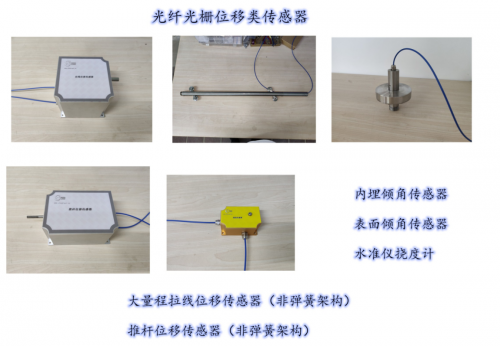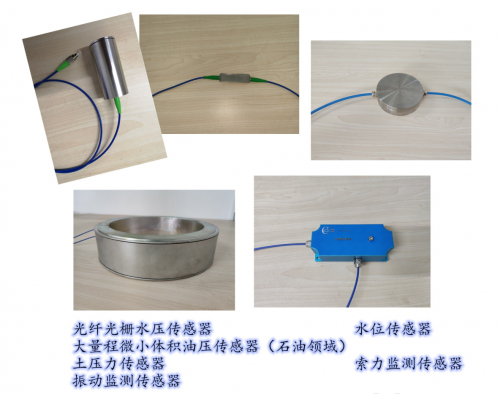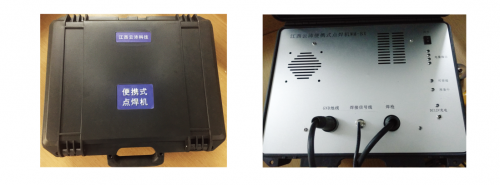# 江西云沛科技 光纤光栅技术助力高低温高压电磁腐蚀环境监测<p segoe="" ui",="" roboto,="" "helvetica="" neue",="" arial,="" "noto="" sans",="" sans-serif,="" "apple="" color="" emoji",="" "segoe="" ui="" symbol",="" emoji";="" white-space:="" normal;="" background-color:="" rgb(255,="" 255,="" 255);="" text-align:="" left;"="" style="font-family: " microsoft="" yahei";="" font-size:="" medium;="" box-sizing:="" border-box;="" margin-top:="" 0px;="" margin-bottom:="" 1rem;="" color:="" rgb(33,="" 37,="" 41);"="">云沛科技,国际国内领先的光纤传感、雷达监测技术、监测设备开发生产集成商,将携手中国工程界打造最先进、最可靠、最高效的工程监测技术手段,并将新技术带出国门,造福全人类,开创中国品牌引领世界新天地。

版权及免责声明：凡本网所属版权作品，转载时须获得授权并注明来源“环球光伏网”，违者本网将保留追究其相关法律责任的权力。凡转载文章，不代表本网观点和立场。